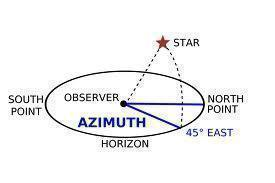•• Azimuth

Azimuth is a measurement at an angle used in the spherical coordinate system. To find a point of interest, the observer creates a vector that is then projected in a perpendicular fashion onto a reference plane. The resulting angle between the projected vector and the reference vector located on the reference plane is the azimuth. It is the direction on the reference plane that is used to determine where the point of interest lies in the spherical coordinate system.

A common use of the azimuth measurement is to find the measurement for the position of a star or other celestial body. The star will be the point of interest and the reference plane will be the horizon or a body of water’s surface. The reference vector point can be designated to a known position such as true north. The azimuth measurement is taken from the angle between the north reference point and the perpendicular projection of the star onto the reference plane, being either the horizon or a body of water.

The measurements of azimuth are done in angular degrees (from 0° to 360°). Azimuth has several practical applications such as navigation, astronomy, engineering, mapping, mining, artillery, and determining lunar and solar rising and setting.Navigation on land denotes azimuth as the lowercase Greek letter alpha. It is the horizontal angle that is measured in a clockwise fashion from a north base line or meridian. It can also be a horizontal angle measurement taken from a clockwise fixed reference plane. An established base direction line can also be used if a fixed reference plane is not easily determined.

General navigation applications use true north as the reference plane starting point. True north is measured as 0° azimuth and other angular measurement systems such as gradian turn units or milliradians can be used instead of degrees. Azimuth cannot exceed the maximum units within the circle reference plane. The maximum in degrees is 359 degrees with 59 arc minutes and 59 arc seconds commonly written as 359° 59′ 59″.

If moving clockwise on the 360° reference plane circles, the azimuth of 90° would be the direction of due east, 180° would be south, and 270° would be west from true north as the reference plane starting point. If a different starting point is used, it must be clearly defined so that everyone using the system is able to accurately locate the point of reference with the correct azimuth measurement.

Azimuth Measurements Based on True North

When using true north as the starting point for the reference plane, the measurement of the azimuth will be 0° or 360°. Moving in a clockwise direction, north northeast is at 22.5°, northeast at 45°, east northeast at 67.5°, east at 90°, east southeast at 112.5°, southeast at 135°, south southeast at 157.5°, south at 180°, south southwest at 202.5°, southwest at 225°, west southwest at 247.5°, west at 270°, west northwest at 292.5°, northwest at 315° and north northwest at 337.5 only to return back to north at 0° or 360°. Azimuth can be located anywhere on the circular reference plane with a defined starting point.

Deriving Azimuth Mathematically

To calculate the azimuth, a few values must be known. If one is standing at a latitude φ1 and longitude zero, one will want to find the azimuth from one’s viewpoint to Point 2 at latitude φ2 and longitude L (positive eastward). By assuming that the earth is a sphere, one can then calculate the azimuth of point of interest in the spherical coordinate system.

The equation looks like the following:

tan(azimuth)=(sin L)/[(cos φ1)(tan φ2)-(sin φ1)(cos L)]

Mapping via Azimuth

Geologists and surveyors can use a geo compass to easily measure azimuth with the designated markings for azimuth. A geo compass is a useful tool to simplify having to do the calculation to figure out an azimuth angle.

Azimuthal map projections help to preserve the azimuths from a central point. Any direction can be set as the plane of reference when using an azimuthal map projection, but it needs to be defined so that others using these measurements are able to correctly determine the azimuth direction via the same system.

Astronomical Azimuths

Celestial navigation uses azimuths as a celestial body’s direction from the observer. Azimuth is generally measured from the north in astronomy but can be done from any direction as long as the direction is defined clearly to have uniformity in the system of users.

Measurement of Right Ascension or Hour Angle

When using the reference plane of, from, and along the celestial equator, the azimuth angles that are derived are called right ascension measurements towards the vernal equinox. If referenced on the celestial meridian, the azimuth angles are called hour angles.

Celestial Navigation and Satellite Dish Horizontal Coordinate

Azimuth is one of the integral units used in the horizontal coordinate system for celestial navigation and satellite dish installation. The azimuth is measured as the angle of the point of interest around the horizon. The other coordinate used in this system is altitude or elevation above the horizon.

Polar Coordinate Systems

Three dimensional polar coordinate systems use azimuths as a measurement of the angle between a positive x axis and the projection of the vector onto the XY plane. It is used to find measurements between the pole, polar axis, and radial coordinate.

281 queries in 0.486 seconds.